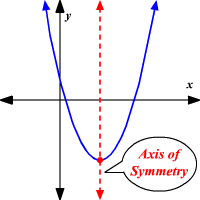# how to find axis of symmetry equation | WTBBLUE

Axis of Symmetry of a Parabola

The graph of a quadratic function is a parabola. The axis of symmetry of a parabola is a vertical line that divides the parabola into two congruent halves. The axis of symmetry always passes through the vertex of the parabola . The x -coordinate of the vertex is the equation of the axis of symmetry of the parabola.For a quadratic function in standard form, y = a x 2 + b x + c , the axis of symmetry is a vertical line x = − b 2 a .